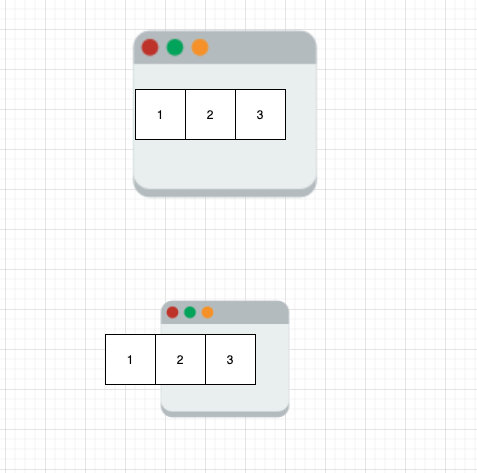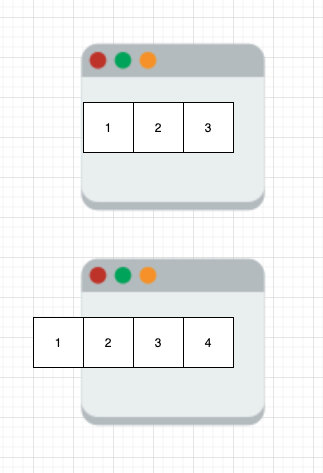## 常见套路

• 固定窗口大小
• 窗口大小不固定，求解最大的满足条件的窗口
• 窗口大小不固定，求解最小的满足条件的窗口（上面的 209 题就属于这种）

## 固定窗口大小

1. l 初始化为 0
2. 初始化 r，使得 r - l + 1 等于窗口大小
3. 同时移动 l 和 r
4. 判断窗口内的连续元素是否满足题目限定的条件
• 4.1 如果满足，再判断是否需要更新最优解，如果需要则更新最优解
• 4.2 如果不满足，则继续。## 可变窗口大小

1. l 和 r 都初始化为 0
2. r 指针移动一步
3. 判断窗口内的连续元素是否满足题目限定的条件
• 4.1 如果满足，再判断是否需要更新最优解，如果需要则更新最优解。并尝试通过移动 l 指针缩小窗口大小。循环执行 4.1
• 4.2 如果不满足，则继续。## 模板代码

``````class Solution:
def minSubArrayLen(self, s: int, nums: List[int]) -> int:
l = total = 0
ans = len(nums) + 1
for r in range(len(nums)):
total += nums[r]
while total >= s:
ans = min(ans, r - l + 1)
total -= nums[l]
l += 1
return  0 if ans == len(nums) + 1 else ans``````